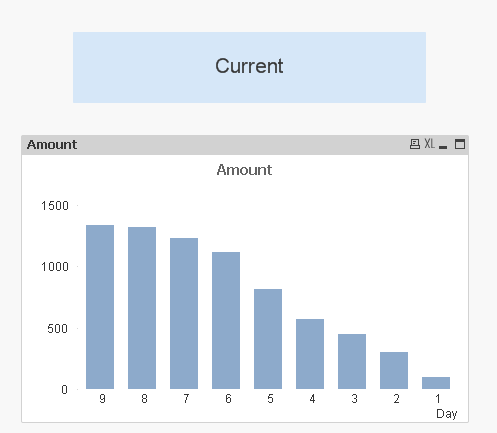# QlikView App Dev

Discussion Board for collaboration related to QlikView App Development.

Announcements
Our next Qlik Insider session will cover new key capabilities. Join us August 11th REGISTER TODAY
cancel
Showing results for
Did you mean:Specialist III

## Reverse accumulation

Hi Experts,

I have made on bar chart , in which

Dimension : Day (1,2,3,4,5,6,7,... so on ) -------  Sorting order is ascending

Expression : sum(amount)

Full accumulation option is check in

Now i have changed the dimension sorting order to descending .

Now my chart is look like.I just want , amount at day one should be at 9th day (like reverse).

Also attching the example file .

1 Solution

Accepted SolutionsMVP

Try with RowNo() instead of Day

=RangeSum(Below(Sum({<Date= {">=\$(=Min([Month Start Date]))<=\$(=Date)"}> }[ERP Amount USD])/1000000,0,RowNo()))

Also make sure to add Date function around Min in your set analysis unless you date is a number.

5 RepliesMVP

Try this:

RangeSum(Below(sum(Amount), 0, Day))Specialist III
Author

I have tried your expression in my actual application , like below

=Rangesum(below(sum({<Date= {">=\$(=min([Month Start Date]))<=\$(=Date)"}> }[ERP Amount USD])/1000000,0,Day))

But it is showing amount at 9th day onlyMVP

Try with RowNo() instead of Day

=RangeSum(Below(Sum({<Date= {">=\$(=Min([Month Start Date]))<=\$(=Date)"}> }[ERP Amount USD])/1000000,0,RowNo()))

Also make sure to add Date function around Min in your set analysis unless you date is a number.MVP

RowNo() will not accumulate to the bottom line:

=RangeSum(Below(Sum({<Date= {">=\$(=Min([Month Start Date]))<=\$(=Date)"}> }[ERP Amount USD])/1000000,0,NoOfRows()) )

or

=RangeSum(Below(Sum({<Date= {">=\$(=Min([Month Start Date]))<=\$(=Date)"}> }[ERP Amount USD])/1000000,0,(NoOfRows()-RowNo()+1)))Specialist III
Author

Thanks Sunny ,

I have tried in this way

=RangeSum(Below(Sum({<Date= {">=\$(=Min([Month Start Date]))<=\$(=Date)"}> }[ERP Amount USD])/1000000,0,)Sum({<Date= {">=\$(=Min([Month Start Date]))<=\$(=Date)"}> }Day))

It is also working ,

Am i doing correct ?Community Browser